# Maxwell-Boltzmann Distribution

01/01/1869View on timelineFigure 1: Gas Movement 

The Maxwell-Boltzmann Distribution

The Maxwell-Boltzmann statistic describes the velocity distribution of the particles that compose a gas at a certain temperature in the thermodynamic equilibrium. The Maxwell-Boltzmann distribution is represented by Figure 2.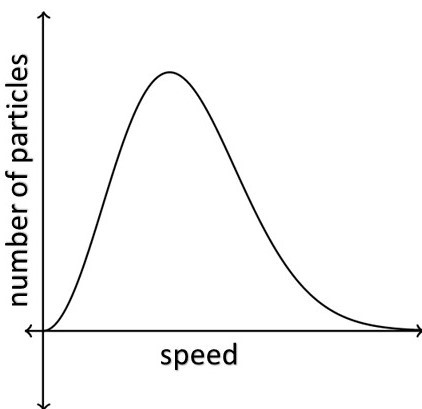Figure 2: The Mazwell-Boltzmann Distribution 

The y-axis represents the number of molecules per unit of speed. So if the graph is higher in a certain region it means that there are more molecules moving at that speed.

The study of Maxwell-Boltzmann distribution began in the 1857 paper “On the Nature of the Motion which we Call Heat” by German physicist Rudolf Clausius, in which the speeds of atoms were calculated.

James Maxwell, In 1860, published “On the Dynamical Theory of Gases”, demonstrated using kinetic theory how to obtain physical properties of gases from the distribution of velocities, taking into account not simply the average speed of the atoms, but their distribution of speeds greater or smaller than the average. Ludwig Boltzmann, In 1868, published a more convincing physical explanation for the Maxwell formula had derived, showing that Maxwell's formula correctly predicted how the number of atoms or molecules with a particular energy would change.

To obtain the velocity distribution of Maxwell-Boltzmann it is possible to use the formalism of the grand canonical ensemble.

The Maxwell-Boltzmann Distribution In The Grand Canonical Ensemble

The grand canonical ensemble It is a way of modeling a thermodynamic system with fixed temperature and fixed chemical potential. For this is used the grand canonical partition function, given by:Onde:

β =1/Kb T, T is the temperature and Kb is the Boltzmann´s constant;

ϵj is the energy of the j-th state;

μ is the chemical potential.

The thermodynamic number of particles is: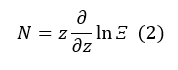And the average value of particles in the j-th state is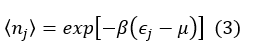As in the grand canonical ensemble the chemical potential is fixed, we define the fugacity of the system as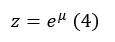and equation 1 becomes: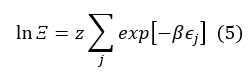so,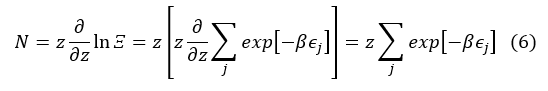and equation 2 becomes: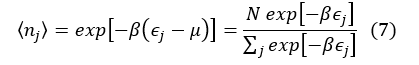which is the discrete form of the classical Maxwell-Boltzmann distribution.

In the thermodynamic limit (N → ∞, V → ∞, N / V = constant), the summation becomes an integral. Knowing that the transformation of a sum into an integral is given by: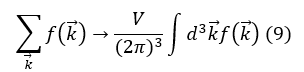And for free particles, in the absence of spin terms in the Hamiltonian operator, the continuous energy spectrum is given by: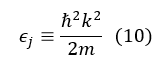and equation 8 becomes:Making p=mv=ℏk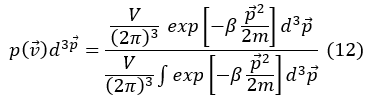Using Gaussian integral, that is: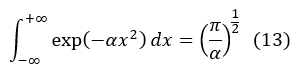And in 3 dimensions: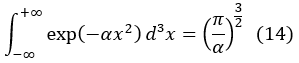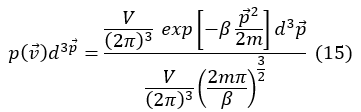Then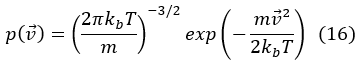Due to the isotropy of the space of the molecular speeds, that is, to the fact that all the properties of the space are the same in all the directions, we can write in spherical coordinates: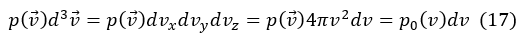so,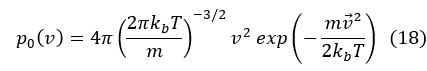It is the probability density of the molecular speed modules.

Speed values can be extracted from the Maxwell-Boltzmann speed distribution function.

The most probable velocity (vmp): corresponds to the maximization of p0 (v).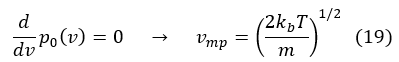The average speed <v> is defined as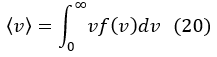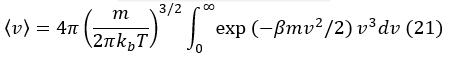using the result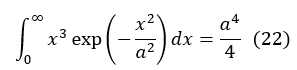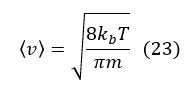The mean square velocity is defined as:using the result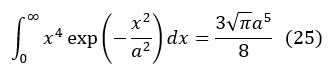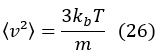and the root mean square (vrms) isgraphically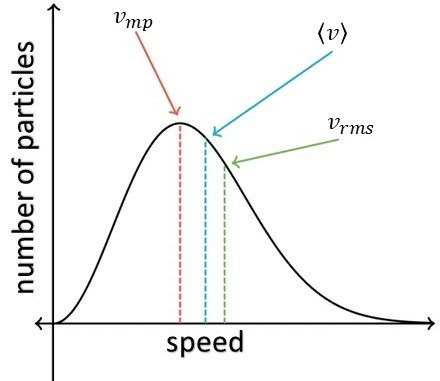Figure 3: Maxwell-Boltzmann speed distribution 

The energy of an ideal gas

The average energy of a molecule of an ideal gas is: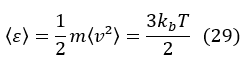Since there are N molecules, the ideal gas energy is:From the graph of the distribution of Maxwell-Boltzmann can extract other information as:

The total area under the entire curve is equal to the total number of molecules in the gas (Figure 4).

The higher the peak height in the Maxwell-Boltzmann distribution graph the lower the average temperature of the molecules that compose the gas. In addition, the higher the gas temperature shifted to the right of the x-axis the graph will be (Figure 5). this happens so that the area under the curve (total number of molecules) remains constant.Figure 4: Total number of perticles .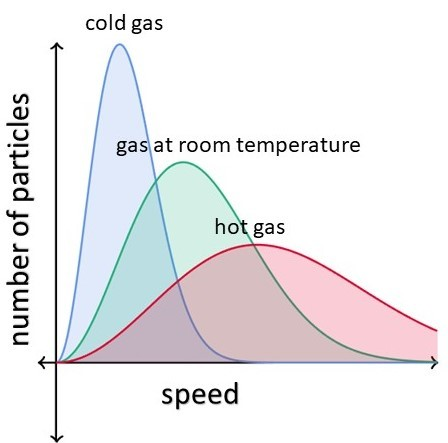Figure 5: Gas Temperature in Maxwell-Boltzmann distributionPHYS 251 Lecture 09 Maxwell-Boltzmann Distribution

References

SALINAS S. R. A. Introduction to Statistical Physics, 2a Ed., Edusp, São Paulo (2013)

 TEIXEIRA, Alessandra de Souza et al. Estudo dos Gases. Available in: <http://processostermodina.wixsite.com/termodinamica/estudo-dos-gases>. Accessed in: 01/14/2019.

 KHAN ACADEMY. What is the Maxwell-Boltzmann distribution?. Available in: <https://www.khanacademy.org/science/physics/thermodynamics/temp-kinetic-theory-ideal-gas-law/a/what-is-the-maxwell-boltzmann-distribution>. Accessed in: 01/14/2019.

Nature motion we call heat - Clausius (1857)

0 comments

Comment

### No comments avaliable.

Author

Info

Published in 14/01/2019

Updated in 19/02/2021

All events in the topic Classical Physics:

Invalid DateMaxwell-Boltzmann Distribution
Invalid DateCathode Rays